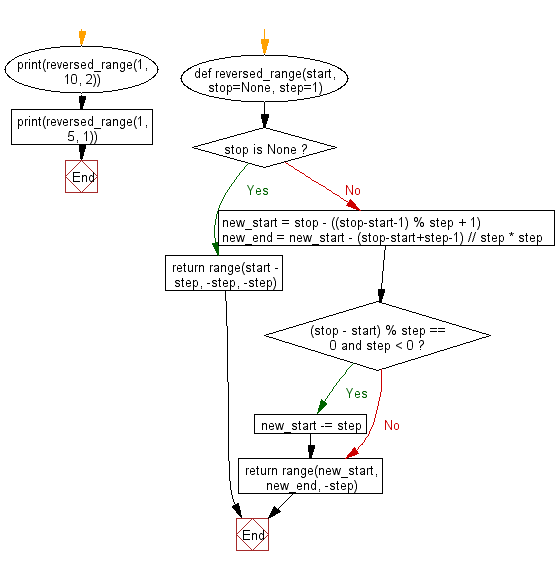﻿ Python Math: Reverse a range - w3resource# Python Math: Reverse a range

## Python Math: Exercise-71 with Solution

Write a Python program to reverse a range.

Sample Solution:-

Python Code:

``````#https://gist.github.com/fcicq/ddb746042150b4e959e6
def reversed_range(start, stop=None, step=1):
if stop is None:
return range(start - step, -step, -step)
else:
new_start = stop - ((stop-start-1) % step + 1)
new_end = new_start - (stop-start+step-1) // step * step
if (stop - start) % step == 0 and step < 0: new_start -= step
return range(new_start, new_end, -step)

print(reversed_range(1, 10, 2))

print(reversed_range(1, 5, 1))
```
```

Sample Output:

```range(9, -1, -2)
range(4, 0, -1)
```

Flowchart:## Visualize Python code execution:

The following tool visualize what the computer is doing step-by-step as it executes the said program:

Python Code Editor:

Have another way to solve this solution? Contribute your code (and comments) through Disqus.

What is the difficulty level of this exercise?

Test your Python skills with w3resource's quiz

﻿

## Python: Tips of the Day

Python: Unpack a Tuple

```>>> items = (0, 'b', 'one', 10,  11, 'zero')
>>> a, b, c, d, e, f = items
>>> print(f)
zero
>>> a, *b, c = items
>>> print(b)
['b', 'one', 10, 11]
>>> *_, a, b = items
>>> print(a)
11
```